Next: Propagation in a conductor Up: Electromagnetic radiation Previous: Dielectric constant of a

Consider a high frequency electromagnetic wave propagating, along the-axis, through a plasma with a longitudinal equilibrium magnetic field,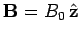. The equation of motion of an individual electron making up the plasma takes the form(1166)

where the first term on the right-hand side is due to the wave electric field, and the second to the equilibrium magnetic field. (As usual, we can neglect the wave magnetic field, provided that the electron motion remains non-relativistic.) Of course,, where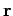is the electron displacement from its equilibrium position. Suppose that all perturbed quantities vary with time like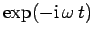, whereis the wave frequency. It follows that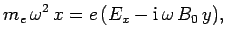(1167)(1168)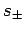(1169)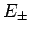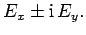(1170)

Using these new variables, Eqs. (1167) and (1168) can be rewritten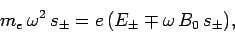(1171)

which can be solved to give(1172)

where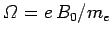is the so-called cyclotron frequency (i.e., the characteristic gyration frequency of free electrons in the equilibrium magnetic field--see Sect. 3.7).

In terms of, the electron displacement can be written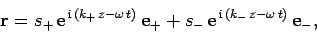(1173)

where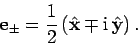(1174)

Likewise, in terms of, the wave electric field takes the form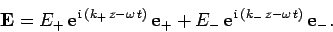(1175)

Obviously, the actual displacement and electric field are the real parts of the above expressions. It follows from Eq. (1175) that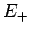corresponds to a constant amplitude electric field which rotates anti-clockwise in the-plane (looking down the-axis) as the wave propagates in the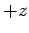-direction, whereas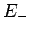corresponds to a constant amplitude electric field which rotates clockwise. The former type of wave is termed right-hand circularly polarized, whereas the latter is termed left-hand circularly polarized. Note also that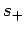and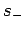correspond to circular electron motion in opposite senses. With these insights, we conclude that Eq. (1172) indicates that individual electrons in the plasma have a slightly different response to right- and left-hand circularly polarized waves in the presence of a longitudinal magnetic field.

Following the analysis of Sect. 9.7, we can deduce from Eq. (1172) that the dielectric constant of the plasma for right- and left-hand circularly polarized waves is(1176)

respectively. Hence, according to Eq. (1154), the dispersion relation for right- and left-hand circularly polarized waves becomes(1177)

respectively. In the limit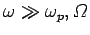, we obtain(1178)

where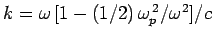and. In other words, in a magnetized plasma, right- and left-hand circularly polarized waves of the same frequency have slightly different wave-numbers

Let us now consider the propagation of a linearly polarized electromagnetic wave through the plasma. Such a wave can be constructed via a superposition of right- and left-hand circularly polarized waves of equal amplitudes. So, the wave electric field can be written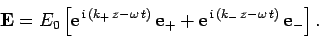(1179)

It can easily be seen that at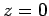the wave electric field is aligned along the-axis. If right- and left-hand circularly polarized waves of the same frequency have the same wave-number (i.e., if) then the wave electric field will continue to be aligned along the-axis as the wave propagates in the-direction: i.e., we will have a standard linearly polarized wave. However, we have just demonstrated that, in the presence of a longitudinal magnetic field, the wave-numbers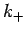and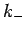are slightly different. What effect does this have on the polarization of the wave?

Taking the real part of Eq. (1179), and making use of Eq. (1178), and some standard trigonometrical identities, we obtain(1180)

The polarization angle of the wave (which is a convenient measure of its plane of polarization) is given by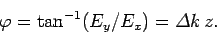(1181)

Thus, we conclude that in the presence of a longitudinal magnetic field the polarization angle rotates as as the wave propagates through the plasma. This effect is known as Faraday rotation. It is clear, from the above expression, that the rate of advance of the polarization angle with distance travelled by the wave is given by(1182)

Hence, a linearly polarized electromagnetic wave which propagates through a plasma with a (slowly) varying electron number density,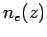, and longitudinal magnetic field,, has its plane of polarization rotated through a total angle(1183)

Note the very strong inverse variation ofwith.

Pulsars are rapidly rotating neutron stars which emit regular blips of highly polarized radio waves. Hundreds of such objects have been found in our galaxy since the first was discovered in 1967. By measuring the variation of the angle of polarization,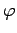, of radio emission from a pulsar with frequency,, astronomers can effectively determine the line integral of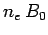along the straight-line joining the pulsar to the Earth using formula (1183). Here,is the number density of free electrons in the interstellar medium, whereas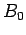is the parallel component of the galactic magnetic field. Obviously, in order to achieve this, astronomers must make the reasonable assumption that the radiation was emitted by the pulsar with a common angle of polarization,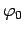, over a wide range of different frequencies. By fitting Eq. (1183) to the data, and then extrapolating to large, it is then possible to determine, and, hence, the amount,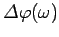, through which the polarization angle of the radiation has rotated, at a given frequency, during its passage to Earth.Next: Propagation in a conductor Up: Electromagnetic radiation Previous: Dielectric constant of a
Richard Fitzpatrick 2006-02-02